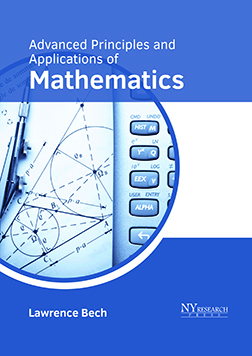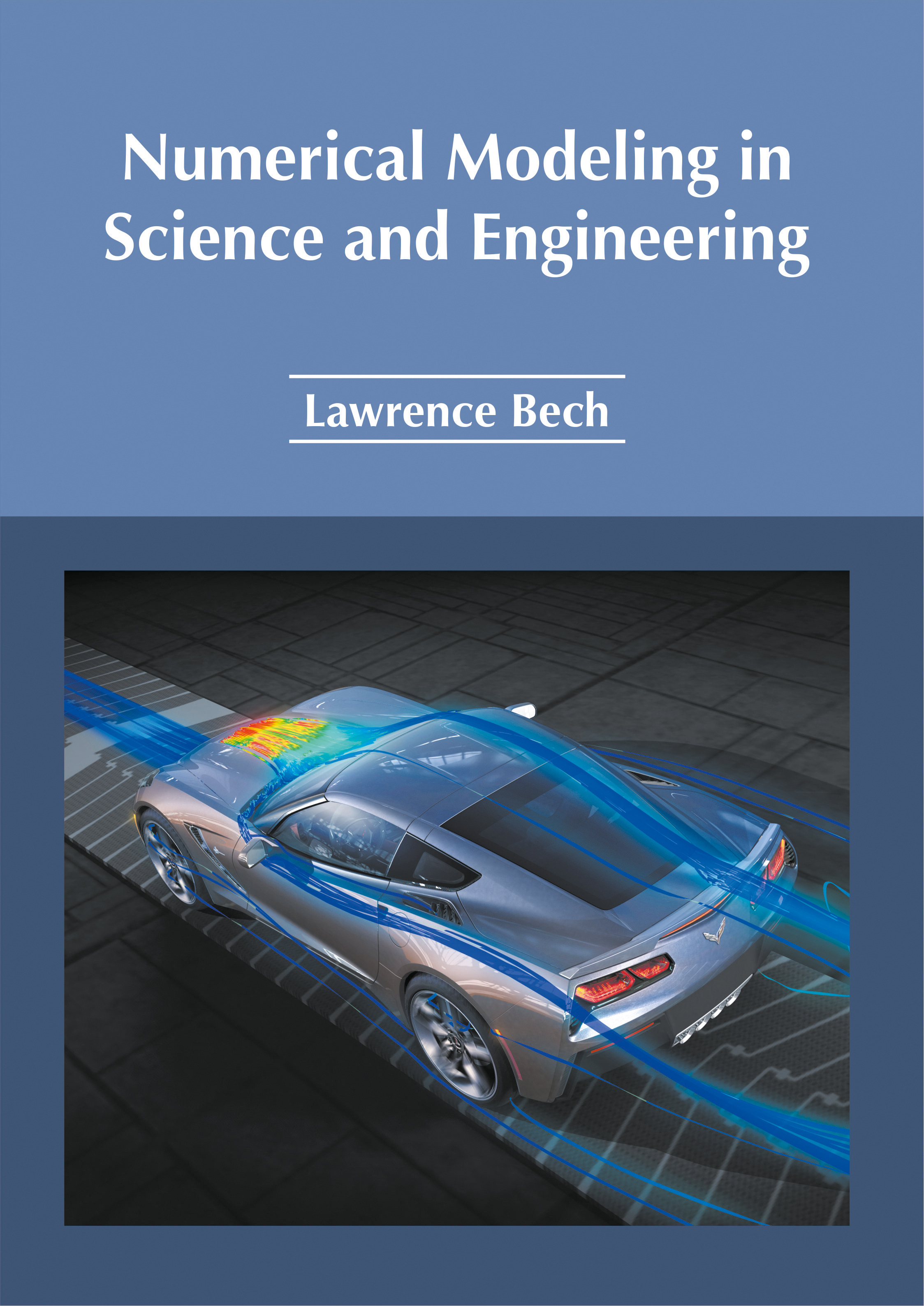BROWSE BY

#### Author

Results 1 - 2 of 2Advanced Principles and Applications of Mathematics Author : Lawrence Bech Subject : Mathematics ISBN :9781632385819 The application of mathematics in various fields such as science and engineering is known as applied mathematics. It studies and works upon practical problems by studying and understanding mathematical Read MoreNumerical Modeling in Science and Engineering Author : Lawrence Bech Subject : Engineering & Technology ISBN :9781632385420 Numerical modeling is the use of computational and mathematical methods to solve scientific problems. This book on numerical modeling in science and engineering deals with the basic elements that are dealt Read More
Results 1 - 2 of 2